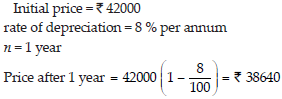Comparing Quantities (Mathematics) Class 8 - NCERT Questions
Q 1.

Find the ratio of the following. (A) Speed of a cycle 15 km per hour to the speed of scooter 30 km per hour.
(B) 5 m to 10 km.
(C) 50 paise to Rs. 5.

SOLUTION: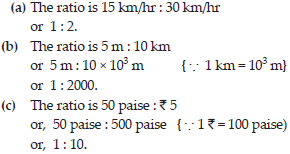Q 2.

Convert the following ratios to percentages.
(A) 3 : 4 (B) 2 : 3.

SOLUTION: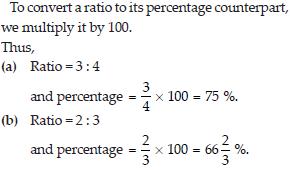Q 3.

72% of 25 students are good in mathematics. How many are not good in mathematics?

SOLUTION: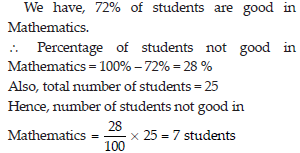Q 4.

A football team won 10 matches out of the total number of matches they played. If their win percentage was 40, then how many matches did they play in all?

SOLUTION: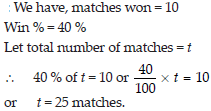Q 5.

If Chameli had Rs. 600 left after spending 75% of her money, how much did she have in the beginning?

SOLUTION: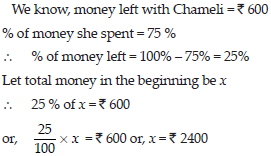Q 6.

If 60% people in a city like cricket, 30% like football and the remaining like other games, then what percent of the people like other games? If the total number of people are 50 lakh, find the exact number who like each type of game.

SOLUTION: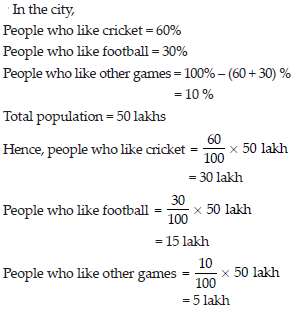Q 7.

A man got a 10% increase in his salary. If his new salary is Rs. 1,54,000, find his original salary.

SOLUTION: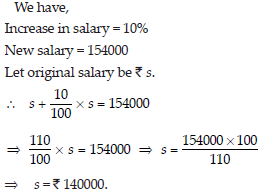Q 8.

On Sunday 845 people went to the Zoo. On Monday only 169 people went. What is the percent decrease in the people visiting the Zoo on Monday?

SOLUTION: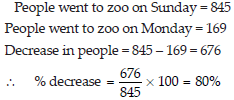Q 9.

A shopkeeper buys 80 articles for Rs. 2,400 and sells them for a profit of 16%. Find the selling price of one article.

SOLUTION: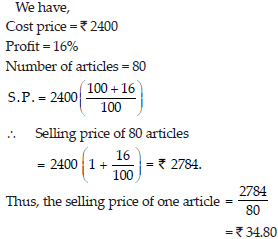Q 10.

The cost of an article was Rs. 15,500. Rs. 450 were spent on its repairs. If it is sold for a profit of 15%, find the selling price of the article.

SOLUTION: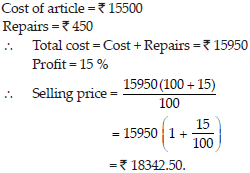Q 11.

A VCR and TV were bought for Rs. 8000 each. The shopkeeper made a loss of 4% on the VCR and a profit of 8% on the TV. Find the gain or loss percent on the whole transaction.

SOLUTION: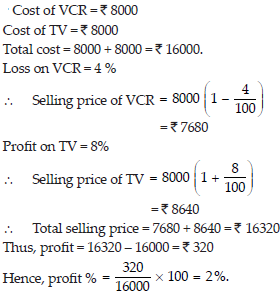Q 12.

During a sale, a shop offered a discount of 10% on the marked prices of all the items. What would a customer have to pay for a pair of jeans marked at Rs. 1450 and two shirts marked at Rs. 850 each?

SOLUTION: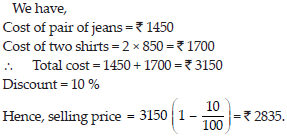Q 13.

A milkman sold two of his buffaloes for Rs. 20,000 each. On one he made a gain of 5% and on the other a loss of 10%. Find his overall gain or loss. (Hint : Find C.P. of each).

SOLUTION: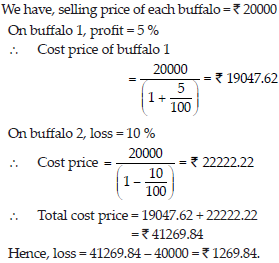Q 14.

The price of a TV is Rs. 13000. The sales tax charged on it is at the rate of 12%. Find the amount that Vinod will have to pay if he buys it.

SOLUTION: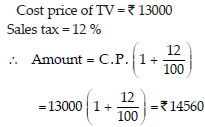Q 15.

Arun bought a pair of skates at a sale where the discount given was 20%. If the amount he pays is Rs. 1,600, find the marked price.

SOLUTION: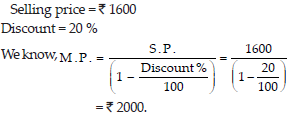Q 16.

I purchased a hair-dryer for Rs. 5,400 including 8% VAT. Find the price before VAT was added.

SOLUTION: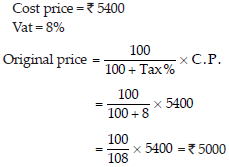Q 17.

Calculate the amount and compound interest on
(A) Rs. 10,800 for 3 years at 1212%per annum compounded annually.
(B) Rs. 18,000 for 212 years at 10% per annum compounded annually.
(C) Rs. 62,500 for 112 years at 8% per annum compounded half yearly.
(D) Rs. 8,000 for 1 year at 9% per annum compounded half yearly.
(E) Rs. 10,000 for 1 year at 8% per annum compounded half yearly.

SOLUTION: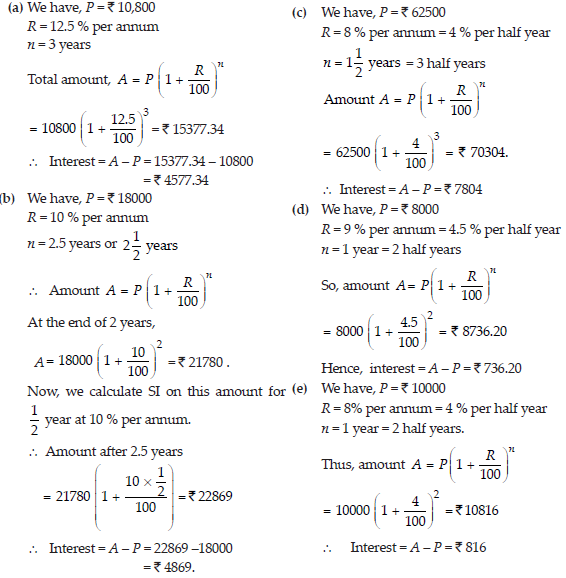Q 18.

Kamla borrowed Rs. 26,400 from a bank to buy a scooter at a rate of 15% per annum compounded yearly. What amount will she pay at the end of 2 years and 4 months to clear the loan?
(Hint : Find A for 2 years with interest is compounded yearly and then find SI on the 2nd year amount for 4/12 years).

SOLUTION: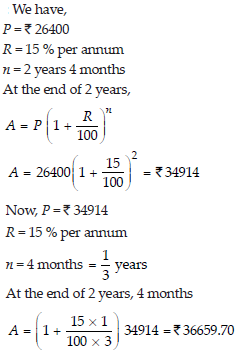Q 19.

Fabina borrows Rs. 12,500 at 12% per annum for 3 years at simple interest and Radha borrows the same amount for the same time period at 10% per annum, compounded annually. Who pays more interest and by how much?

SOLUTION: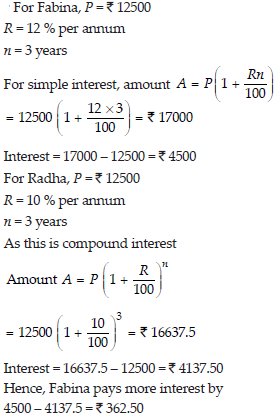Q 20.

I borrowed Rs. 12,000 from Jamshed at 6% per annum simple interest for 2 years. Had I borrowed this sum at 6% per annum compound interest, what extra amount would I have to pay?

SOLUTION: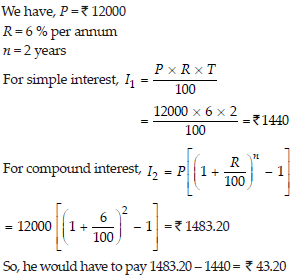Q 21.

Vausudevan invested Rs. 60,000 at an interest rate of 12% per annum compounded half yearly. What amount would he get
(i) after 6 months? (ii) after 1 year?

SOLUTION: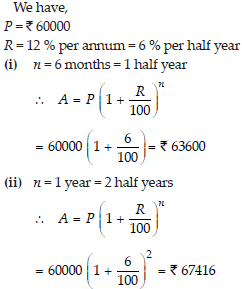Q 22.

Arif took a loan of Rs. 80,000 from a bank. If the rate of interest is 10% per annum, find the difference in amounts he would be paying after 11/2 years if the interest is

SOLUTION: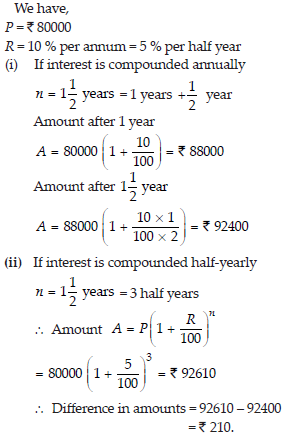Q 23.

Maria invested Rs. 8,000 in a business. She would be paid interest at 5% per annum compounded annually. Find
(i) The amount credited against her name at the end of the second year.
(ii) The interest for the 3rd year.

SOLUTION: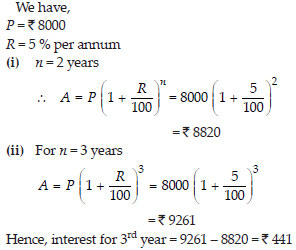Q 24.

Find the amount of the compound interest on Rs. 10,000 for 11/2 years at 10% per annum, compounded half yearly. Would this interest be more than the interest he would get if it was compounded annually?

SOLUTION: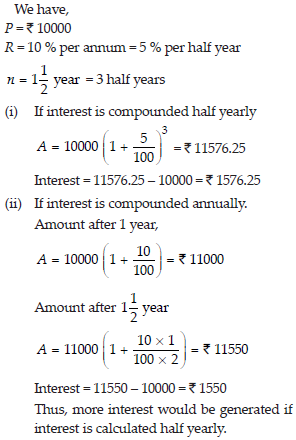Q 25.

Find the amount which Ram will get on Rs. 4096, if he gave it for 18 months at 121/2% per annum, interest being compounded half yearly.

SOLUTION: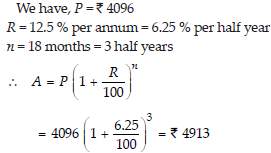Q 26.

The population of a place increased to 54,000 in 2003 at a rate of 5% per annum (i) find the population in 2001. (ii) what would be its population in 2005?

SOLUTION: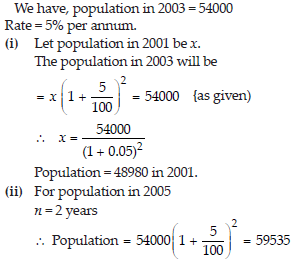Q 27.

In a laboratory, the count of bacteria in a certain experiment was increasing at the rate of 2.5% per hour. Find the bacteria at the end of 2 hours if the count was initially 5,06,000.

SOLUTION: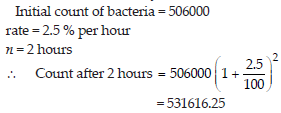Q 28.

A scooter was bought at Rs. 42,000. Its value depreciated at the rate of 8% per annum. Find its value after one year.

SOLUTION: